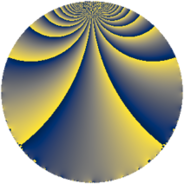# Properties

 Label 80.2.lLevel $80$ Weight $2$ Character orbit 80.l Rep. character $\chi_{80}(21,\cdot)$ Character field $\Q(\zeta_{4})$ Dimension $16$ Newform subspaces $1$ Sturm bound $24$ Trace bound $0$

# Related objects

## Defining parameters

 Level: $$N$$ $$=$$ $$80 = 2^{4} \cdot 5$$ Weight: $$k$$ $$=$$ $$2$$ Character orbit: $$[\chi]$$ $$=$$ 80.l (of order $$4$$ and degree $$2$$) Character conductor: $$\operatorname{cond}(\chi)$$ $$=$$ $$16$$ Character field: $$\Q(i)$$ Newform subspaces: $$1$$ Sturm bound: $$24$$ Trace bound: $$0$$

## Dimensions

The following table gives the dimensions of various subspaces of $$M_{2}(80, [\chi])$$.

Total New Old
Modular forms 28 16 12
Cusp forms 20 16 4
Eisenstein series 8 0 8

## Trace form

 $$16q - 4q^{4} - 12q^{6} + O(q^{10})$$ $$16q - 4q^{4} - 12q^{6} + 4q^{10} - 8q^{11} - 12q^{12} + 4q^{14} - 8q^{15} + 16q^{16} - 8q^{19} + 8q^{20} - 20q^{22} + 8q^{24} - 16q^{26} + 24q^{27} - 4q^{28} - 16q^{29} + 16q^{34} - 4q^{36} - 16q^{37} + 20q^{38} + 60q^{42} + 8q^{43} + 40q^{44} - 4q^{46} - 40q^{47} - 40q^{48} - 16q^{49} - 4q^{50} - 32q^{51} + 56q^{52} + 16q^{53} + 32q^{54} + 16q^{56} - 12q^{58} - 8q^{59} - 28q^{60} + 16q^{61} - 8q^{62} + 40q^{63} - 16q^{64} + 40q^{67} - 48q^{68} + 16q^{69} - 8q^{70} - 40q^{72} - 72q^{74} + 16q^{77} - 16q^{78} + 16q^{79} + 16q^{80} - 16q^{81} - 76q^{82} + 40q^{83} - 64q^{84} - 16q^{85} + 28q^{86} + 36q^{90} + 32q^{91} - 52q^{92} - 48q^{93} - 36q^{94} + 32q^{95} + 8q^{96} + 60q^{98} - 8q^{99} + O(q^{100})$$

## Decomposition of $$S_{2}^{\mathrm{new}}(80, [\chi])$$ into newform subspaces

Label Dim. $$A$$ Field CM Traces $q$-expansion
$$a_2$$ $$a_3$$ $$a_5$$ $$a_7$$
80.2.l.a $$16$$ $$0.639$$ $$\mathbb{Q}[x]/(x^{16} - \cdots)$$ None $$0$$ $$0$$ $$0$$ $$0$$ $$q-\beta _{9}q^{2}+(\beta _{3}-\beta _{6}+\beta _{11})q^{3}+(\beta _{1}+\cdots)q^{4}+\cdots$$

## Decomposition of $$S_{2}^{\mathrm{old}}(80, [\chi])$$ into lower level spaces

$$S_{2}^{\mathrm{old}}(80, [\chi]) \cong$$ $$S_{2}^{\mathrm{new}}(16, [\chi])$$$$^{\oplus 2}$$